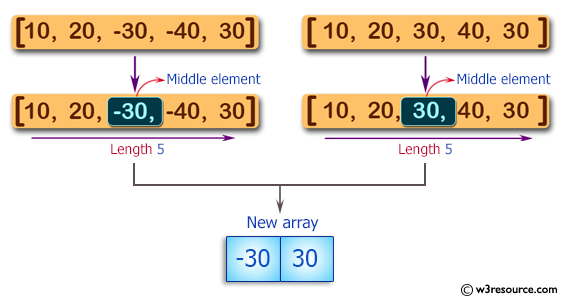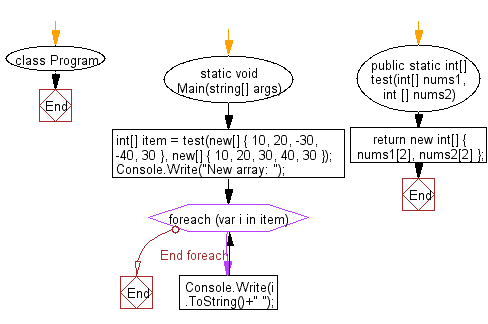﻿ C# Sharp exercises: Create a new array containing the middle elements from the two given arrays of integers, each length 5 - w3resource# C# Sharp Basic Algorithm Exercises: Create a new array containing the middle elements from the two given arrays of integers, each length 5

## C# Sharp Basic Algorithm: Exercise-95 with Solution

Write a C# Sharp program to create a new array containing the middle elements from the two given arrays of integers, each length 5.

Pictorial Presentation:Sample Solution:-

C# Sharp Code:

``````using System;
namespace exercises
{
class Program
{
static void Main(string[] args)
{
int[] item = test(new[] { 10, 20, -30, -40, 30 }, new[] { 10, 20, 30, 40, 30 });

Console.Write("New array: ");

foreach(var i in item)
{
Console.Write(i.ToString()+" ");
}
}
public static int[]  test(int[] nums1, int [] nums2)
{
return new int[] { nums1, nums2 };
}
}
}
```
```

Sample Output:

`New array: -30 30`

Flowchart:C# Sharp Code Editor:

Improve this sample solution and post your code through Disqus

What is the difficulty level of this exercise?

﻿

New Content: Composer: Dependency manager for PHP, R Programming